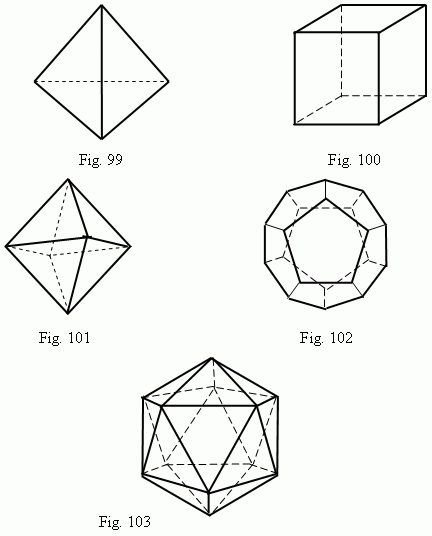# Regular polyhedrons

Regular polygons. Convex regular polyhedrons: tetrahedron,
hexahedron (cube), octahedron, dodecahedron, icosahedron.
Non-convex regular polyhedrons.

A polyhedron is called a regular one, if all its faces are equal regular polygons and the same number of faces join in each its vertex. It is known only five convex regular polyhedrons and four non-convex regular polyhedrons. The regular convex polyhedrons are the following: a tetrahedron ( 4 faces, Fig.99 ); a hexahedron ( 6 faces, Fig.100 ) – well known for us as a cube ; an octahedron ( 8 faces, Fig.101 ); a dodecahedron (12 faces, Fig.102); an icosahedron ( 20 faces, Fig.103 ).It is possible to inscribe a sphere into any regular polyhedron and to circumscribe a sphere around any regular polyhedron.